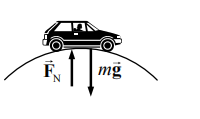### Solution Found!# Physics Behind Normal Force: Car & Driver on a Hill's Rounded Top at S

Chapter 5, Problem 14

(choose chapter or problem)

Get Unlimited Answers! Check out our subscriptions
QUESTION:

A sports car of mass 950 kg (including the driver) crosses the rounded top of a hill (radius = 95 m) at 22 m/s. Determine

(a) the normal force exerted by the road on the car.

(b) the normal force exerted by the car on the 72-kg driver, and

(c) the car speed at which the normal force on the driver equals zero.

##### Physics Behind Normal Force: Car & Driver on a Hill's Rounded Top at SWant To Learn More? To watch the entire video and ALL of the videos in the series:

Unpack the concept of normal force on a sports car and its driver atop a hill. Utilize Newton's second law to calculate these forces based on speed and hill curvature. Discover the speed at which the driver feels weightless in the car.

QUESTION:

A sports car of mass 950 kg (including the driver) crosses the rounded top of a hill (radius = 95 m) at 22 m/s. Determine

(a) the normal force exerted by the road on the car.

(b) the normal force exerted by the car on the 72-kg driver, and

(c) the car speed at which the normal force on the driver equals zero.

Step 1 of 4

The mass of the car with the driver is, m = 950 kg.

The radius of the hill is, $$r = 95 m$$.

The speed of the car is, $$v = 22 m/s$$.

The mass of the driver is, $$m_d = 72\ \text{kg}$$ .

The free body diagram of the car at the instant, when it is at the top, is: